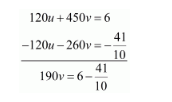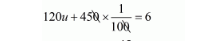# Ramesh travels 760 km to his home partly by train and partly by car.Question:

Ramesh travels 760 km to his home partly by train and partly by car. He takes 8 hours if he travels 160 km. by train and the rest  by car. He takes 12 minutes more if the travels 240 km by train and the rest by car. Find the speed of the train and car respectively.

Solution:

Let the speed of the train be x km/hour that of the car be y km/hr, we have the following cases

Case I: When Ramesh travels 760 Km by train and the rest by car

Time taken by Ramesh to travel $160 \mathrm{Km}$ by train $=\frac{160}{x} h r s$

Time taken by Ramesh to travel $(760-160)=600 \mathrm{Km}$ by car $=\frac{600}{y} h r s$

Total time taken by Ramesh to cover $760 \mathrm{Km}=\frac{160}{x}+\frac{600}{y}$

It is given that total time taken in 8 hours

$\frac{160}{x}+\frac{600}{y}=8$

$8\left(\frac{20}{x}+\frac{75}{y}\right)=8$

$\left(\frac{20}{x}+\frac{75}{y}\right)=\frac{8}{8}$

$\frac{20}{x}+\frac{75}{y}=1 \cdots(i)$

Case II: When Ramesh travels 240Km by train and the rest by car

Time taken by Ramesh to travel $240 \mathrm{Km}$ by train $=\frac{240}{x} \mathrm{hrs}$

Time taken by Ramesh to travel $(760-240)=520 \mathrm{Km}$ by car $=\frac{520}{y} h r s$

In this case total time of the journey is 8 hours 12 minutes

$\frac{240}{x}+\frac{520}{y}=8 h r s 12 \mathrm{~min}$ utes

$\frac{240}{x}+\frac{520}{y}=8 \frac{12}{60}$

$\frac{240}{x}+\frac{520}{y}=\frac{41}{5}$

$40\left(\frac{6}{x}+\frac{13}{y}\right)=\frac{41}{5}$

$\frac{6}{x}+\frac{13}{y}=\frac{41}{5} \times \frac{1}{40}$

$\frac{6}{x}+\frac{13}{y}=\frac{41}{200}$ $\ldots$ (ii)

Putting $\frac{1}{x}=u$ and, $\frac{1}{y}=u$, the equations $(i)$ and $(i i)$ reduces to

$20 u+75 v=1 \cdots($ iii $)$

$6 u+13 v=\frac{41}{200} \cdots(i v)$

Multiplying equation (iii) by 6 and (iv)by 20 the above system of equation becomes

$120 u+450 v=6 \cdots(v)$

$120 u+260 v=\frac{41}{10} \cdots(v i)$

Subtracting equation (vi) from (v) we get$190 v=\frac{60-41}{10}$

$190 v=\frac{19}{10}$

$v=\frac{19}{10} \times \frac{1}{190}$

$v=\frac{1}{100}$

Putting $v=\frac{1}{100}$ in equation $(v)$, we get$120 u+\frac{45}{10}=6$

$120 u=6-\frac{45}{10}$

$120 u=\frac{60-45}{10}$

$120 u=\frac{15}{10}$

$u=\frac{15}{10} \times \frac{1}{120}$

$u=\frac{1}{80}$

Now

$u=\frac{1}{80}$

$\frac{1}{x}=\frac{1}{80}$

$x=80$

and

$v=\frac{1}{100}$

$\frac{1}{y}=\frac{1}{100}$

$y=100$

Hence, the speed of the train is $80 \mathrm{~km} / \mathrm{hr}$,

The speed of the car is $100 \mathrm{~km} / \mathrm{hr}$